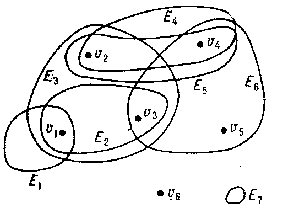# Hypergraph

A generalization of the concept of a graph. A hypergraph is defined by a set $V$, whose elements are known as vertices, and by a family ${\mathcal E}$ of subsets of $V$, known as edges or hyperedges. A hypergraph is denoted by $( V, {\mathcal E} )$. The concept of a hypergraph is a variant of the familiar concepts of a complex, a block design and a network.

Two vertices of a hypergraph are said to be adjacent if there exists an edge containing these vertices. A vertex $v$ and an edge $E$ of a hypergraph are said to be incident if $v \in E$. A hypergraph $H$ with $n$ vertices and $m$ edges may be defined by an incidence matrix, i.e. by the matrix $\| a _ {ij} \|$ of dimension $n \times m$ in which the columns correspond to the edges while the rows correspond to the vertices of the hypergraph, and where

$$a _ {ij} = \ \left \{ \begin{array}{ll} 1 &\textrm{ if } v _ {i} \in E _ {j} , \\ 0 &\textrm{ if } v _ {i} \notin E _ {j} , \\ \end{array} \ \ i = 1 \dots n; \ j = 1 \dots m. \right .$$

It is possible to assign to each matrix $M$ consisting of zeros and ones a hypergraph for which $M$ is the incidence matrix. The hypergraph $H ^ {*}$ is called the dual of the hypergraph $H$ if the incidence matrix of $H ^ {*}$ is obtained by transposing the incidence matrix of $H$. The number of edges of a hypergraph $H$ that are incident to a given vertex is called the degree of the vertex. The degree of an edge is the number of vertices of the hypergraph incident to this edge. A hypergraph $( V ^ { \prime } , {\mathcal E} ^ { \prime } )$ is called a subhypergraph of a hypergraph $( V, {\mathcal E} )$ if $V ^ { \prime } \subseteq V$, ${\mathcal E} ^ { \prime } \subseteq {\mathcal E}$, and if a vertex $v$ from $V ^ { \prime }$ and an edge $E$ from ${\mathcal E} ^ { \prime }$ are incident in the hypergraph $( V ^ { \prime } , {\mathcal E} ^ { \prime } )$ if and only if they are incident in the hypergraph $( V, {\mathcal E} )$.

A hypergraph may be represented in a plane by identifying the vertices of the hypergraph with points of the plane and by identifying the edges with connected domains containing the vertices incident with these edges. For instance, it is possible to represent the hypergraph $H$ with set of vertices $V = \{ v _ {1} \dots v _ {6} \}$ and family of edges

$${\mathcal E} = \{ E _ {1} = \{ v _ {1} \} ,\ E _ {2} = \{ v _ {1} , v _ {3} \} ,\ E _ {3} = \{ v _ {1} , v _ {2} , v _ {3} \} ,$$

$${} E _ {4} = E _ {5} = \{ v _ {2} , v _ {4} \} ,\ E _ {6} = \{ v _ {3} , v _ {4} , v _ {5} \} , E _ {7} = \emptyset \}$$

in a plane, as shown in the figure.Figure: h048470a

A hypergraph $H$ may be represented by a bipartite graph (cf. Graph, bipartite) $K( H)$ in which the vertices of one part $U _ {1}$ correspond to the vertices of the hypergraph, while the vertices of the other part $U _ {2}$ correspond to the edges of $H$. Two vertices $u ^ \prime$ from $U _ {1}$ and $u ^ {\prime\prime}$ from $U _ {2}$ will be connected by an edge in the graph $K( H)$ if the vertex of the hypergraph corresponding to $u ^ \prime$ is incident to the edge of the hypergraph corresponding to $u ^ {\prime\prime}$. A hypergraph is a graph if each of its edges has degree two. An important special case of the concept of a "hypergraph" is that of a matroid. Many concepts in the theory of graphs, such as connectedness, planarity, the chromatic number, and the external and internal stability numbers, may be applied to hypergraphs, as may many theorems that are applicable to graphs.

How to Cite This Entry:
Hypergraph. Encyclopedia of Mathematics. URL: http://encyclopediaofmath.org/index.php?title=Hypergraph&oldid=47300
This article was adapted from an original article by A.A. Sapozhenko (originator), which appeared in Encyclopedia of Mathematics - ISBN 1402006098. See original article